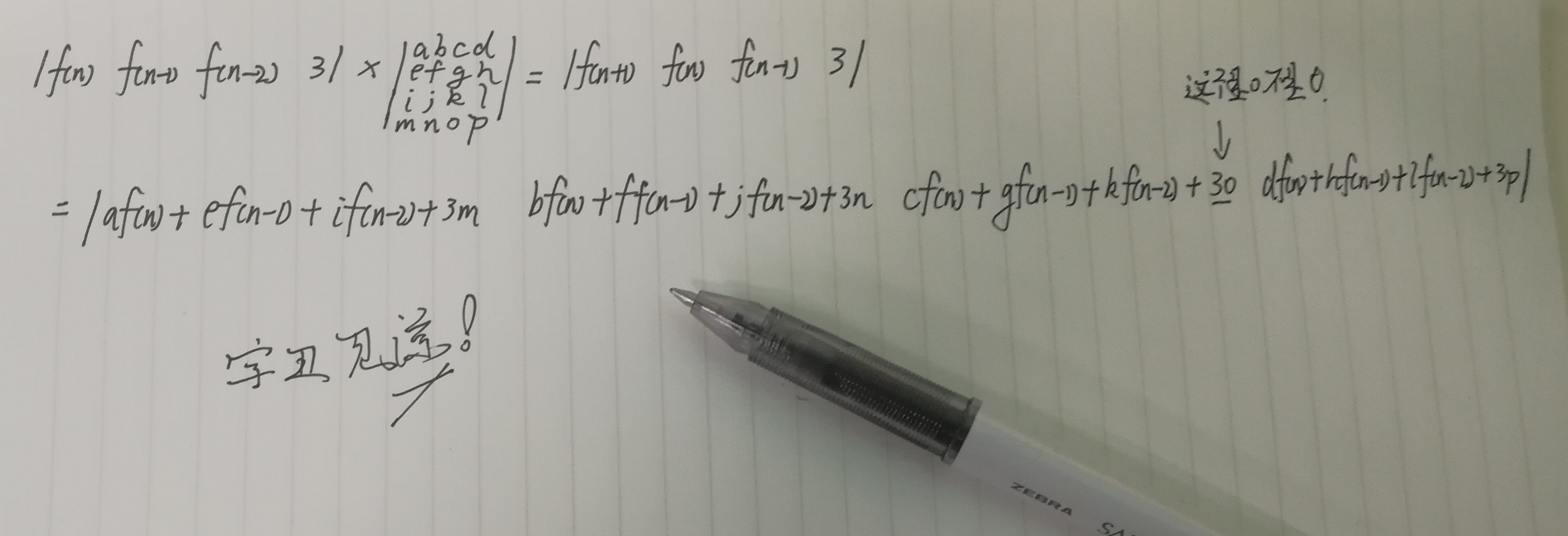# 浅谈矩阵[简洁易懂]——上篇

——正片开始——

1. 矩阵加法：

2. 矩阵乘法：

$$C_{i,j}=\sum_{k=1}^{n}a_{i,k}b_{k,j}$$

struct Matrix{
int a[N][N];
Matrix(){memset(a,0,sizeof a);}
int ln,lm;//长度
Matrix operator *(const Matrix&b)const{
Matrix res;res.ln=ln;res.lm=b.lm;
for(int i=1;i<=ln;i++)
for(int j=1;j<=b.lm;j++)
for(int k=1;k<=lm;k++)
res.a[i][j]=(res.a[i][j]+a[i][k]*b.a[k][j])%MOD;
}
};

$F(n)= \left|\begin{matrix} f(n) & f(n-1) \end{matrix} \right|$

F(n)包含了f(n)和f(n-1)两个信息，正是我们得到f(n+1)所需要的。

（ans矩阵是1*2的，base矩阵得要是2*2的，才能得到1*2的ans矩阵）。

$$\left|\begin{matrix} f(n) & f(n-1) \end{matrix} \right| \times \left|\begin{matrix} a & b \\ c & d \end{matrix} \right| = \left|\begin{matrix} af(n)+cf(n-1) & bf(n)+df(n-1))\end{matrix} \right| = \left|\begin{matrix} f(n+1) & f(n) \end{matrix} \right|$$

ll qpow(int x,int y){
ll tmp=x,con=1;
while(y){
if(y&1)con=con*tmp%MOD;
tmp=tmp*tmp%MOD;
y>>=1;
}return con;
}

$F(n)= \left|\begin{matrix} f(2) & f(1) \end{matrix} \right| \times \left|\begin{matrix} 1 & 1 \\ 1 & 0 \end{matrix} \right|$

#include<bits/stdc++.h>
using namespace std;
long long n;
const long long mod=1e9+7;
struct Matrix{//封装成结构体更方便食用
long long a;
Matrix(){memset(a,0,sizeof(a));}
Matrix operator *(const Matrix &b)const{
Matrix res;
for(int i=1;i<=2;i++)
for(int j=1;j<=2;j++)
for(int k=1;k<=2;k++)
res.a[i][j]=(res.a[i][j]+a[i][k]*b.a[k][j])%mod;
return res;
}
}ans,base;
void init(){//初始化ans和base矩阵
base.a=base.a=base.a=1;
ans.a=ans.a=1;
}
void qpow(long long b){//矩阵快速幂
while(b){
if(b&1)ans=ans*base;
base=base*base;
b>>=1;
}
}
int main(){
scanf("%lld",&n);
if(n<=2){
puts("1");return 0;
}
init();
qpow(n-2);
printf("%lld",ans.a%mod);
return 0;
}

（我为啥要给自己挖这么大一个坑...），这待会儿Latex要写死我$F(3)= \left| \begin{matrix} f(3) & f(2) & f(1) & 3 \end{matrix} \right|$

update：忘了给代码了，补上

#include<bits/stdc++.h>
using namespace std;
int data=0,w=1;char ch=0;
while(ch!='-' && (ch<'0'||ch>'9'))ch=getchar();
if(ch=='-')w=-1,ch=getchar();
while(ch>='0' && ch<='9')data=data*10+ch-'0',ch=getchar();
return data*w;
}
const long long mod=1000000007ll;
struct Matrix{
long long a;
Matrix(){memset(a,0,sizeof a);}
Matrix operator *(const Matrix&b)const{
Matrix res;
for(int i=1;i<=4;i++)
for(int j=1;j<=4;j++)
for(int k=1;k<=4;k++)
res.a[i][j]=(res.a[i][j]+a[i][k]*b.a[k][j])%mod;
return res;
}
}ans,base;
void qpow(long long b){
while(b){
if(b&1)ans=ans*base;
base=base*base;
b>>=1;
}
}
void init(){
//f=3,f=12,f=45
ans.a=45;ans.a=12;ans.a=3;ans.a=3;
base.a=base.a=base.a=base.a=base.a=1;
base.a=3;base.a=2;
}
int main(){
if(n==1)
return puts("3"),0;
else if(n==2)
return puts("12"),0;
else if(n==3)
return puts("45"),0;
else{
init();
qpow(n-3);
printf("%lld",ans.a%mod);
}
return 0;
} 

posted @ 2019-11-11 20:50  LightHouseOfficial  阅读(...)  评论(... 编辑 收藏